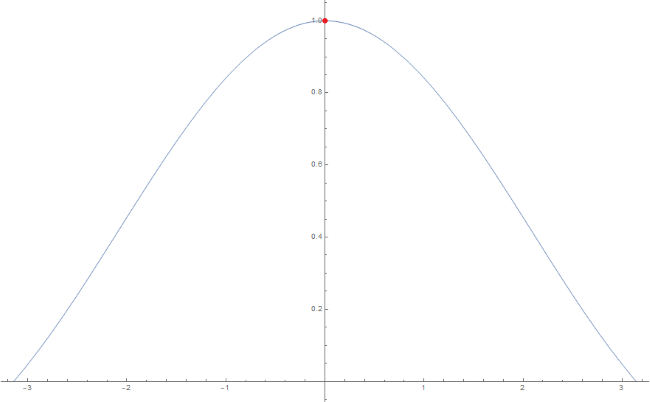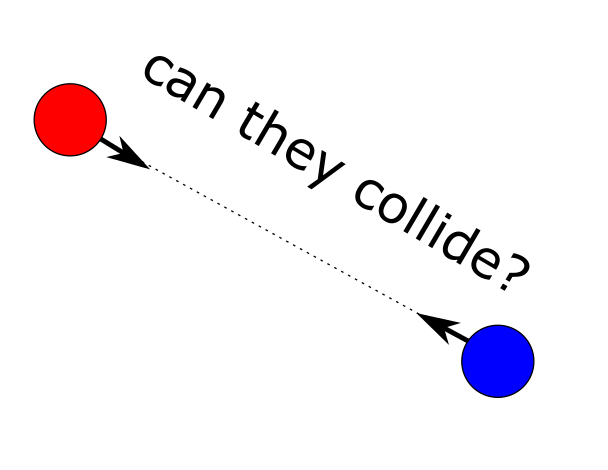# Research diaries #2: Singularities and regularizations

in StemSociallast year (edited)

A lot of my research work is somewhat technical/abstract. There is a connection between the theory and the application but the questions are usually defined from the perspective of theory. A question I often deal with is whether something is well-defined. I will explain this within the framework of singularities and regularizations. There is a cute photo of my cat at the end :D

A large part of applied math is concerned with making the reasoning in experimental subjects rigorous. A subpart is concerned with developing theory for singularities. Singularities here mean that somewhere you are doing an illegal operation like dividing by zero. The corresponding math is then concerned with extending the system with the singularity to a system without singularity.

## The basic idea

These singularity problems are in a sense very similar to the limit problems which you come across in a fundamental calculus course. So let's stary with an example. Below you can see the graph of the function sin(x)/x:It does not exist at x =0, but I can make it defined at zero by saying that at x=0 it is equal to 1 since if I approach zero from positive or negative x it appears to go to 1. This idea results in the definition of the limit. So for this example x=0 is a singularity. Specifically, it is called a removable singularities since we can extend the definition of sin(x)/x by fixing sin(x)/x at x=0. This yields a regularized equation for sin(x)/x.

## The actual problem and the solution

This concept can be extended to more complicated systems. Systems which play an important role in the science subjects are differential equations. Informally, these are systems where governing equations can also depend on infinitesimal change of temporal or spatial variables. For example, for planetary motions the force between two bodies is proportional to 1/distance^2 (up to scaling constants). This means that if planets collide the governing equations are not defined. Technicalities on regularizations for the n-body problem can be found hereSo we need some kind of limit which regularizes the system. There are two ways to proceed with these type of problems:

1. You can prove that solutions of these singular systems have a regularized form, just as with the sin(x)/x case,

2. or you can regularize the governing equations themselves and then apply standard theory to show the existence of the desired solution.

Usually you don't only want to prove that there exists a regularized solutions. You want additional properties of the underlying solutions. So I find that (1) generally doesn't allow you to apply standard theory when you have regularized the system. This is because standard theory usually only is available in a setting where the governing equations do not contain singularities. So generally, I opt for method (2). If you expect that there is no theory for the regularized form you can opt for (1).

## My research work

Singularities and their regularizations have been a somewhat common theme in my research work. For most of my applications they are the result of a global transformation which gives the governing equations an overall nice form but at some points singularties pop up which you have to deal with. Only after having regularized the system I can apply standard techniques to prove the existence of the desired solutions. Hence, this regularization step is just a subproblem which kicks off the main problem.

Hope you enjoyed my somewhat cryptical mathematics post. Will try to pick something with pretty pictures next time :P

Anyway here is a cute picture of my cat:

Cat taxSort:

The cat works for me :)

She is my chief inspector :D

I like to keep a cat nearby while I do math. Not sure why, but I think it helps me concentrate.

It is true they are distraction absorbers

hello murder mittens

she only murders my cables connectivity

last year (edited)

This time, I won't comment on the cat (too much data in it).

2 . or you can regularize the governing equations themselves and then apply standard theory to show the existence of the desired solution.

Does this include changing the governing equations by possibly very different ones? For instance, we may have a primary system of equations that apply in a given domain or under certain circumstances. During the course of the evolution, we may however leave the domain of definition of the primary system, so that the whole set of equations need to be replaced by something else.

Of course, I have physics in mind. For instance, Newtonian mechanics needs to be upgraded to special relativity for fastly moving systems. In this case however, we can recover the former by taking the right limit of the latter.

During the course of the evolution, we may however leave to domain of definition of the primary system, so that the whole set of equations need to be replaced by something else.

In a sense this is what I do but then in a local sense. At the singularity the equations are not defined so I construct regularized equations which are actually like a new set of equation which can be used in a neighbourhood of the singularity.

So I have something like a state space manifold where I have a special chart in the neighbourhood of the singularity.

Oh I see. So it is really like the analytical continuation of the sinc function that you took as an example. Thanks for answering!

An example of these class of problems would be:

under what conditions on the parameter a do there exist solutions to

dx/dt= ax/t + bt such that \lim_{t \to 0} x(t)/t^2 exists (where b is a free parameter, which here acts like a perturbation term)

with some straightforward calculus you can show that if a \neq 2 the desired solution exists.

The b-term acts like a perturbation term to the ax/t part so the more general version of the above you would replace the b-term by some very general perturbation.

Ok I (think I) see.

However, in your example, if b=0 then the a \neq 2 condition is not necessary, is it? In this maybe pathological case, the solution is trivially proportional to ta. In this sense, the b-term has in my taste deeper implications than just being a perturbation.

Am I missing something trivial or am I putting too much emphasis on an irrelevant configuration?

For b=0 you have a zero solution. That's correct. Casting it in the more general framework you want to find something that holds for all b. So b defines her a space of functions.

More general you would look at
dx/dt= ax/t + g(x,t)
with g in a specific space. So you would want to find results for all g in that specific space.

These problems are motivated by classical problems of the type:
dx/dt = Ax + f(x)
with f(x) in O(x^2)

As always, I focus too much on the pathological cases. Thanks for the clarifications ;)

cat :3.

What I don´t get about finite time singularities (and I dont know much about math, maybe Im completely wrong), for example those that model financial bubbles, what is the correct term for the curve? People often say "there will be soon a parabolic price move" or "Bitcoin will go parabolic" But to my understanding parabolas are curves that grow much slower (exponential) and don´t reach infinity in finite time? Is it hyperbolic?

I don't know much about finance :3

To get an intuition of that phenomenon you can investigate dx/dt = 1/ (2 x), then the solution is x(t) = sqrt(t-c) (up to potential errors :P) while dx/dt = x is x(t) = c e^t

It's the cat for me. At least, he/she is not abstract. :)Thanks for your contribution to the STEMsocial community. Feel free to join us on discord to get to know the rest of us!

Please consider delegating to the @stemsocial account (80% of the curation rewards are returned).

Please consider including @stemsocial as a beneficiary to get a stronger support.

Your content has been voted as a part of Encouragement program. Keep up the good work!

Use Ecency daily to boost your growth on platform!

Support Ecency
Vote for new Proposal
Delegate HP and earn more